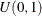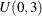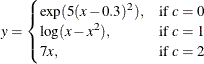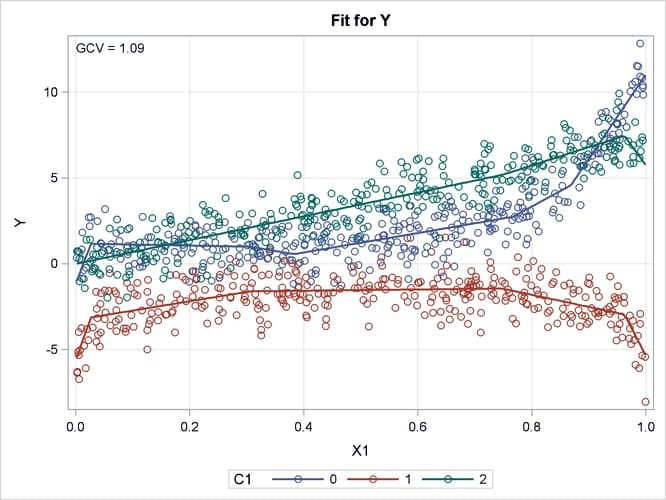# The ADAPTIVEREG Procedure

### Example 25.2 Fitting Data with Mixture Structures

This example shows how you can use PROC ADAPTIVEREG to fit a model from a data set that contains mixture structures. It also demonstrates how to use the CLASS statement.

Consider a simulated data set that contains a response variable and two predictors, one continuous and the other categorical. The continuous predictor is sampled from the uniform distribution, and the classification variable is sampled fromand then rounded to integers. The response variable is constructed from three different models that depend on the CLASS variable levels, with error sampled from the standard normal distribution.The following statements create the artificial data set Mixture:

data Mixture;
drop i;
do i=1 to 1000;
X1 = ranuni(1);
C1 = int(3*ranuni(1));
if C1=0 then Y=exp(5*(X1-0.3)**2)+rannor(1);
else if C1=1 then Y=log(X1*(1-X1))+rannor(1);
else Y=7*X1+rannor(1);
output;
end;
run;


The standard deviation for the response without noise is 3.14. So the response variable Y in the data set Mixture has a signal-to-noise ratio of 3.14. With a classification variable and a continuous variable in the data set, the objective is to fit a nonparametric model that can reveal the underlying three different data-generating processes. The following statements use the ADAPTIVEREG procedure to fit the data:

ods graphics on;
proc adaptivereg data=Mixture plots=fit;
class c1;
model y=c1 x1;
run;


Because the data contain two explanatory variables, graphical presentation of the fitted model is possible. The PLOTS= FIT option in the PROC ADAPTIVEREG statement requests the fit plot. The CLASS statement specifies that C1 is a classification variable.

Output 25.2.1 displays the parameter estimates for the 13 selected basis functions after backward selection. For Basis1, the coefficient estimate is -4.3871. It is constructed from the intercept and the classification variable C1 at levels 0 and 1.

Output 25.2.1: Parameter Estimates

The ADAPTIVEREG Procedure

Regression Spline Model after Backward Selection
Name Coefficient Parent Variable Knot Levels
Basis0 5.1794   Intercept
Basis1 60.2517 Basis0 C1   1 0
Basis3 1.5768 Basis0 C1   0
Basis5 101.33 Basis3 X1 0.7665
Basis6 -88.9774 Basis3 X1 0.7665
Basis7 -76.9359 Basis2 X1 0.7480
Basis8 88.0913 Basis2 X1 0.7480
Basis9 87.5864 Basis4 X1 0.7480
Basis10 -95.0232 Basis4 X1 0.7480
Basis11 -89.5813 Basis1 X1 0.02653
Basis13 11.3393 Basis3 X1 0.3829
Basis15 31.1335 Basis3 X1 0.8687
Basis17 -56.5339 Basis4 X1 0.9624
Basis19 -5.0676 Basis1 X1 0.3060

Output 25.2.2 displays the fitted linear splines overlaid with the original data. PROC ADAPTIVEREG captures the three underlying data-generating processes. For observations with C1 at level 0, the shape of the fitted splines is quite similar to the exponential function. For observations with C1 at level 1, the shape of the fitted spline suggests a symmetric function along X1 with a symmetry point approximately equal to 0.5. The function at each side of the symmetry point is analogous to the logarithmic transformation. For the rest of the observations with C1 at level 2, PROC ADAPTIVEREG suggests a strict linear model. The fitted model is very close to the true model. PROC ADAPTIVEREG fits the model in an automatic and adaptive way, except that it needs the CLASS statement to name the classification variable.

Output 25.2.2: Raw Data and Fitted ModelYou might notice that some basis functions have their parent basis functions not listed in the parameter estimates table (Output 25.2.1). This is because their parent basis functions are dropped during the model selection process. You can view the complete set of basis functions used in the model selection by specifying the DETAILS=BASES option in the PROC ADAPTIVEREG statement, as in the following statements:

proc adaptivereg data=Mixture details=bases;
class c1;
model y=c1 x1;
run;


Output 25.2.3: Basis Function Information

The ADAPTIVEREG Procedure

Basis Information
Name Transformation
Basis0 1
Basis1 Basis0*(C1 = 1 OR C1 = 0)
Basis2 Basis0*NOT(C1 = 1 OR C1 = 0)
Basis3 Basis0*(C1 = 0)
Basis4 Basis0*NOT(C1 = 0)
Basis5 Basis3*MAX(X1 - 0.7665019053,0)
Basis6 Basis3*MAX(0.7665019053 - X1,0)
Basis7 Basis2*MAX(X1 - 0.7480032624,0)
Basis8 Basis2*MAX(0.7480032624 - X1,0)
Basis9 Basis4*MAX(X1 - 0.7480032624,0)
Basis10 Basis4*MAX(0.7480032624 - X1,0)
Basis11 Basis1*MAX(X1 - 0.0265261848,0)
Basis12 Basis1*MAX(0.0265261848 - X1,0)
Basis13 Basis3*MAX(X1 - 0.3829118905,0)
Basis14 Basis3*MAX(0.3829118905 - X1,0)
Basis15 Basis3*MAX(X1 - 0.8686795225,0)
Basis16 Basis3*MAX(0.8686795225 - X1,0)
Basis17 Basis4*MAX(X1 - 0.962359583,0)
Basis18 Basis4*MAX( 0.962359583 - X1,0)
Basis19 Basis1*MAX(X1 - 0.3060246363,0)
Basis20 Basis1*MAX(0.3060246363 - X1,0)

You can produce a SAS DATA step for scoring new observations by using the information provided in the parameter estimate table and the basis information table, as shown in the following statements:

data New;
basis1  = (c1=1 OR c1=0);
basis3  = (c1=1);
basis5  = NOT(c1=1)*MAX(x1-0.7665019053,0);
basis7  = NOT(c1=1 OR c1=0)*MAX(x1-0.7665019053,0);
basis8  = NOT(c1=1 OR c1=0)*MAX(0.7665019053-x1,0);
basis9  = (c1=1)*MAX(x1-0.5530566455,0);
basis10 = (c1=1)*MAX(0.5530566455-x1,0);
basis11 = (c1=1)*MAX(x1-0.045800759,0);
basis13 = (c1=1)*MAX(x1-0.9526330293,0);
basis15 = (c1=1 OR c1=0)*MAX(x1-0.9499325226,0);
basis17 = (c1=1 OR c1=0)*MAX(x1-0.5142821095,0);
basis19 = (c1=1 OR c1=0)*MAX(x1-0.9889635476,0);
pred    = 5.3829 - 4.3871*basis1 + 32.7761*basis3 +
20.2859*basis5 - 11.4183*basis7 - 7.0758*basis8 +
58.4911*basis9 - 71.6388*basis10 - 69.0764*basis11 -
119.71*basis13 + 66.5733*basis15 + 6.6681*basis17 -
185.21*basis19;
run;